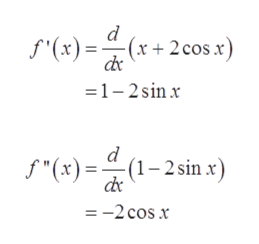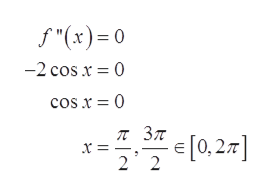# Find the point of inflection of the graph of the function. (If an answer does not exist, enter DNE.)F(x)=x+2cosx, [0, 2pi]smaller x-value (x,y)=lager x-value (x,y)=Describe the concavity. (Enter your answers using interval notation. If an answer does not exist, enter DNE.)concave upward (     )concave downward (     )

Question
10 views

Find the point of inflection of the graph of the function. (If an answer does not exist, enter DNE.)

F(x)=x+2cosx, [0, 2pi]

smaller x-value (x,y)=

lager x-value (x,y)=

Describe the concavity. (Enter your answers using interval notation. If an answer does not exist, enter DNE.)

concave upward (     )

concave downward (     )

check_circle

Step 1

Given,

Step 2

For point of inflection, differentiate function twicehelp_outlineImage Transcriptionclose(x + 2cos x) f"(x) =(x+ 2cos .x) de =1- 2sin x s"(x) = (1–2sin x) de =-2 cos x fullscreen
Step 3

Now, take second derivativ...help_outlineImage Transcriptionclosef"(x)= 0 -2 cos x = 0 cos x = 0 [=zo]>=- [0, 2.7] fullscreen

### Want to see the full answer?

See Solution

#### Want to see this answer and more?

Solutions are written by subject experts who are available 24/7. Questions are typically answered within 1 hour.*

See Solution
*Response times may vary by subject and question.
Tagged in

### Calculus